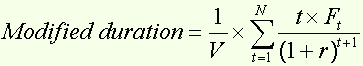Modified duration of a bond

Around the formula...

The modified duration of a bond measures the percentage change in its price for a given change in interest rates. The price of a bond with a modified duration of 4 will increase by 4% when interest rates fall from 7% to 6%, while the price of another bond with a modified duration of 3 will increase by just 3%.

From a mathematical standpoint, modified duration can be defined as the absolute value of the first derivative of a bond's price with respect to interest rates, divided by the price:where r is the market rate and Ft the cash flows generated by the bond.